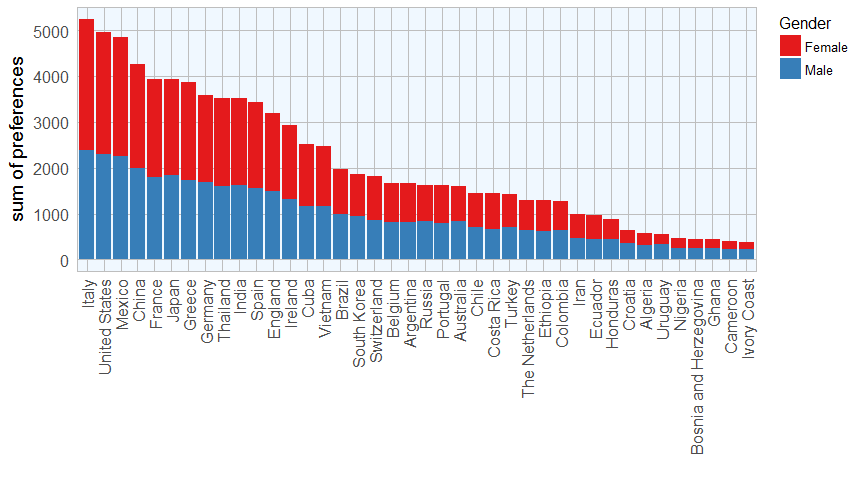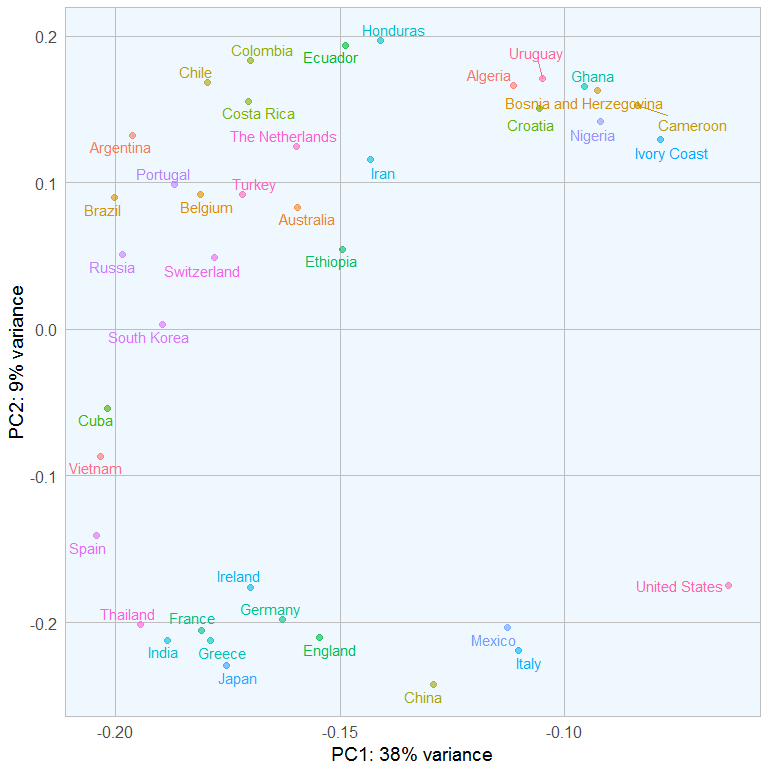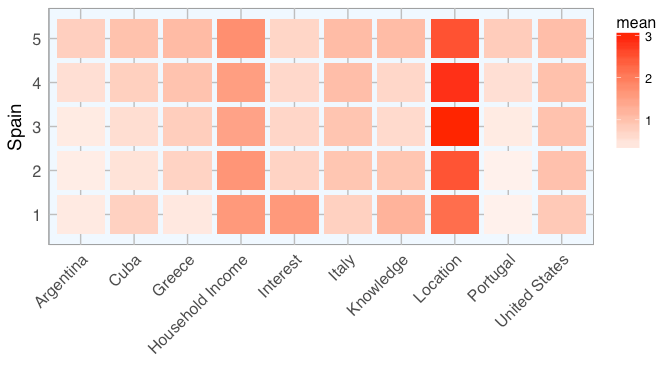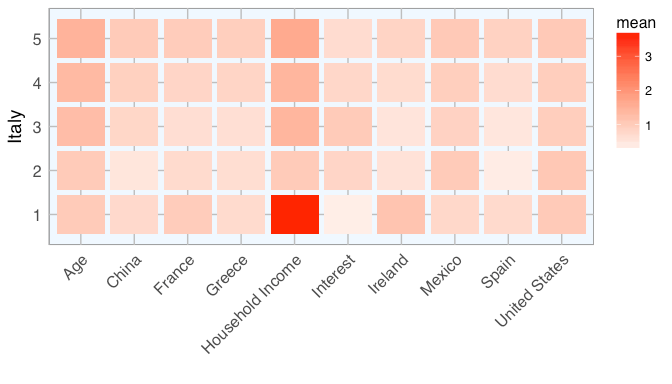Want to share your content on R-bloggers? click here if you have a blog, or here if you don't.

This week I want to show how to run machine learning applications on a Spark cluster. I am using the sparklyr package, which provides a handy interface to access Apache Spark functionalities via R.

The question I want to address with machine learning is whether the preference for a country’s cuisine can be predicted based on preferences of other countries’ cuisines.

### Apache Spark

Apache Spark™ can be used to perform large-scale data analysis workflows by taking advantage of its parallel cluster-computing setup. Because machine learning processes are iterative, running models on parallel clusters can vastly increase the speed of training. Obviously, Spark’s power comes to pass when dispatching it to external clusters, but for demonstration purposes, I am running the demo on a local Spark instance.

#### MLlib

Spark’s distributed machine learning library MLlib sits on top of the Spark core framework. It implements many popular machine learning algorithms, plus many helper functions for data preprocessing. With sparklyr you can easily access MLlib. You can work with a couple of different machine learning algorithms and with functions for manipulating features and Spark dataframes. Additionally, you can also perform SQL queries. sparklyr also implements dplyr, making it especially convenient for handling data.

If you don’t have Spark installed locally, run:

```library(sparklyr)
spark_install(version = "2.0.0")
```

Now we can connect to a local Spark instance:

```library(sparklyr)
sc <- spark_connect(master = "local", version = "2.0.0")
```

### Preparations

Before I start with the analysis, I am preparing my custom ggplot2 theme and load the packages tidyr (for gathering data for plotting), dplyr (for data manipulation) and ggrepel (for non-overlapping text labels in plots).

```library(tidyr)
library(ggplot2)
library(ggrepel)
library(dplyr)

my_theme <- function(base_size = 12, base_family = "sans"){
theme_minimal(base_size = base_size, base_family = base_family) +
theme(
axis.text = element_text(size = 12),
axis.title = element_text(size = 14),
panel.grid.major = element_line(color = "grey"),
panel.grid.minor = element_blank(),
panel.background = element_rect(fill = "aliceblue"),
strip.background = element_rect(fill = "lightgrey", color = "grey", size = 1),
strip.text = element_text(face = "bold", size = 12, color = "black"),
legend.position = "right",
legend.justification = "top",
panel.border = element_rect(color = "grey", fill = NA, size = 0.5)
)
}
```

### The Data

Of course, the power of Spark lies in speeding up operations on large datasets. But because that’s not very handy for demonstration, I am here working with a small dataset: the raw data behind The FiveThirtyEight International Food Association’s 2014 World Cup.

This dataset is part of the fivethirtyeight package and provides scores for how each person rated their preference of the dishes from several countries. The following categories could be chosen:

• 5: I love this country’s traditional cuisine. I think it’s one of the best in the world.
• 4: I like this country’s traditional cuisine. I think it’s considerably above average.
• 3: I’m OK with this county’s traditional cuisine. I think it’s about average.
• 2: I dislike this country’s traditional cuisine. I think it’s considerably below average.
• 1: I hate this country’s traditional cuisine. I think it’s one of the worst in the world.
• N/A: I’m unfamiliar with this country’s traditional cuisine.

Because I think that whether someone doesn’t know a country’s cuisine is in itself information, I recoded NAs to 0.

```library(fivethirtyeight)

food_world_cup[food_world_cup == "N/A"] <- NA
food_world_cup[, 9:48][is.na(food_world_cup[, 9:48])] <- 0
food_world_cup\$gender <- as.factor(food_world_cup\$gender)
food_world_cup\$location <- as.factor(food_world_cup\$location)
```

The question I want to address with machine learning is whether the preference for a country’s cuisine can be predicted based on preferences of other countries’ cuisines, general knowledge and interest in different cuisines, age, gender, income, education level and/ or location.

Before I do any machine learning, however, I want to get to know the data. First, I am calculating the percentages for each preference category and plot them with a pie chart that is facetted by country.

```# calculating percentages per category and country
percentages <- food_world_cup %>%
select(algeria:vietnam) %>%
gather(x, y) %>%
group_by(x, y) %>%
summarise(n = n()) %>%
mutate(Percent = round(n / sum(n) * 100, digits = 2))

# rename countries & plot
percentages %>%
mutate(x_2 = gsub("_", " ", x)) %>%
mutate(x_2 = gsub("(^|[[:space:]])([[:alpha:]])", "\\1\\U\\2", x_2, perl = TRUE)) %>%
mutate(x_2 = gsub("And", "and", x_2)) %>%
ggplot(aes(x = "", y = Percent, fill = y)) +
geom_bar(width = 1, stat = "identity") +
theme_minimal() +
coord_polar("y", start = 0) +
facet_wrap(~ x_2, ncol = 8) +
scale_fill_brewer(palette = "Set3") +
labs(fill = "")
```As we can already see from the distributions, there are big differences between countries. Some countries’ cuisines are very well known and liked (e.g. Italy, Mexico and the United States), while others are only known to a handful of people (e.g. Algeria, Croatia, Ghana, etc.).

#### Imputing missing values

In the demographic information, there are a few missing values. These, I want to impute:

```library(mice)

dataset_impute <- mice(food_world_cup[, -c(1, 2)],  print = FALSE)
food_world_cup <- cbind(food_world_cup[, 2, drop = FALSE], mice::complete(dataset_impute, 1))
```

#### Transforming preference values

Strictly speaking, the preference data is categorical. But because using them as factor levels would make the models much more complex and calculation-intensive, I am converting the factors to numbers and transform them by dividing through the mean of non-zero values for each country.

```food_world_cup[8:47] <- lapply(food_world_cup[8:47], as.numeric)

countries <- paste(colnames(food_world_cup)[-c(1:7)])

for (response in countries) {
food_world_cup[paste(response, "trans", sep = "_")] <- food_world_cup[response] / mean(food_world_cup[food_world_cup[response] > 0, response])
}
```

As we can see by plotting the distribution of transformed values, they are far from being normal distributions. Moreover, we still see big differences between well known and not well known countries - this means that the data is unbalanced.

```food_world_cup %>%
gather(x, y, algeria_trans:vietnam_trans) %>%
mutate(x_2 = gsub("_trans", "", x)) %>%
mutate(x_2 = gsub("_", " ", x_2)) %>%
mutate(x_2 = gsub("(^|[[:space:]])([[:alpha:]])", "\\1\\U\\2", x_2, perl = TRUE)) %>%
mutate(x_2 = gsub("And", "and", x_2)) %>%
ggplot(aes(y)) +
geom_density(fill = "navy", alpha = 0.7) +
my_theme() +
facet_wrap(~ x_2, ncol = 8) +
labs(x = "transformed preference")
```#### Most liked cuisines and gender biases

Then, I wanted to know which countries were the most liked and whether we see a gender bias in preference for some country’s cuisines. The first plot shows the sum of preference values for each country, separated by male and female answers.

```food_world_cup_gather <- food_world_cup %>%
collect %>%
gather(country, value, algeria:vietnam)

food_world_cup_gather\$value <- as.numeric(food_world_cup_gather\$value)
food_world_cup_gather\$country <- as.factor(food_world_cup_gather\$country)
```
```food_world_cup_gather <- food_world_cup_gather %>%
mutate(x_2 = gsub("_", " ", country)) %>%
mutate(x_2 = gsub("(^|[[:space:]])([[:alpha:]])", "\\1\\U\\2", x_2, perl = TRUE)) %>%
mutate(x_2 = gsub("And", "and", x_2))

order <- aggregate(food_world_cup_gather\$value, by = list(food_world_cup_gather\$x_2), FUN = sum)

food_world_cup_gather %>%
mutate(x_2 = factor(x_2, levels = order\$Group.1[order(order\$x, decreasing = TRUE)])) %>%
ggplot(aes(x = x_2, y = value, fill = gender)) +
geom_bar(stat = "identity") +
scale_fill_brewer(palette = "Set1") +
my_theme() +
theme(axis.text.x = element_text(angle = 90, vjust = 0.5, hjust = 1)) +
labs(fill = "Gender",
x = "",
y = "sum of preferences")
```Cuisines from Italy, the United States and Mexico were the most liked, from Ghana, Cameroon and the Ivory Coast were least liked (that they were also less well known playes into it, of course). No country shows an obvious gender bias, though.

To dig a bit deeper into gender differences, I am calculating the differences between mean preference of males and females for each country. Here, I am using the country list that I defined earlier when transforming the values.

Because I want to paste the country list to dplyr’s functions, I need to use standard evaluation (SE). Be default, dplyr uses non-standard evalutaion (NSE), i.e. variable names are given without quotation marks. This makes it possible to easily convert between R and SQL code. But each dplyr function also has a version to use with SE: they each have a “” appended to the function name, here e.g. *mutate_each()*.

```food_world_cup %>%
collect %>%
mutate_each_(funs(as.numeric), countries) %>%
group_by(gender) %>%
summarise_each_(funs(mean), countries) %>%
summarise_each_(funs(diff), countries) %>%
gather(x, y) %>%
mutate(x_2 = gsub("_", " ", x)) %>%
mutate(x_2 = gsub("(^|[[:space:]])([[:alpha:]])", "\\1\\U\\2", x_2, perl = TRUE)) %>%
mutate(x_2 = gsub("And", "and", x_2)) %>%
ggplot(aes(x = x_2, y = y)) +
geom_bar(stat = "identity", fill = "navy", alpha = 0.7) +
my_theme() +
theme(axis.text.x = element_text(angle = 90, vjust = 0.5, hjust = 1)) +
labs(x = "",
y = "difference\nbetween gender")
```All gender differences are very close to zero, so it seems that men and women generally have similar food preferences.

### Spark

Now, that I’m somewhat familiar with the data, I copy it to the Spark instance:

```food_world_cup <- copy_to(sc, food_world_cup)
```

#### Principal Component Analysis (PCA)

One of the functions of Spark’s machine learning functions is for PCA: ml_pca(). I am using it to get an idea about where the countries fall on a 2-dimensional plane of the first two principal components.

```pca <- food_world_cup %>%
mutate_each_(funs(as.numeric), countries) %>%
ml_pca(features = paste(colnames(food_world_cup)[-c(1:47)]))

library(tibble)
as.data.frame(pca\$components) %>%
rownames_to_column(var = "labels") %>%
mutate(x_2 = gsub("_trans", "", labels)) %>%
mutate(x_2 = gsub("_", " ", x_2)) %>%
mutate(x_2 = gsub("(^|[[:space:]])([[:alpha:]])", "\\1\\U\\2", x_2, perl = TRUE)) %>%
mutate(x_2 = gsub("And", "and", x_2)) %>%
ggplot(aes(x = PC1, y = PC2, color = x_2, label = x_2)) +
geom_point(size = 2, alpha = 0.6) +
geom_text_repel() +
labs(x = paste0("PC1: ", round(pca\$explained.variance, digits = 2) * 100, "% variance"),
y = paste0("PC2: ", round(pca\$explained.variance, digits = 2) * 100, "% variance")) +
my_theme() +
guides(fill = FALSE, color = FALSE)
```The least well known countries cluster on the top right, while the most liked are on the bottom right. PC2 seems to be mainly driven by how many 0s there are in the data.

#### Preparing the data for machine learning

First, I need to convert the factor strings of the non-country features into indexes. To do so, I am using the ft_string_indexer() function. For other feature transformation functions, check out sparklyr’s website.

```food_world_cup <- tbl(sc, "food_world_cup") %>%
ft_string_indexer(input_col = "interest", output_col = "interest_idx") %>%
ft_string_indexer(input_col = "gender", output_col = "gender_idx") %>%
ft_string_indexer(input_col = "age", output_col = "age_idx") %>%
ft_string_indexer(input_col = "household_income", output_col = "household_income_idx") %>%
ft_string_indexer(input_col = "education", output_col = "education_idx") %>%
ft_string_indexer(input_col = "location", output_col = "location_idx") %>%
ft_string_indexer(input_col = "knowledge", output_col = "knowledge_idx")
```

Now I can divide the data into training and test sets using the sdf_partition() function.

```partitions <- food_world_cup %>%
sdf_partition(training = 0.75, test = 0.25, seed = 753)
```

#### Modeling

Now, I run the Random Forest algorithm to predict each country’s preference based on all other countries’ preferences and the demographic information. Check back with sparklyr’s website to find out about other machine learning algorithms you can use.

For each country (as response variable), I am defining the features as all other countries’ transformed values and the indexed factor variables.

Then, I am filtering out all data rows where the response variable was 0. When I left the 0s in the data, the countries with many 0s introduced a very strong bias to the prediction. Here, I needed to use standard evaluation again, this time with lazyeval’s interp() function and a formula.

I am using the ml_random_forest() function to run classification models. Inititally, I run a model on all features, then extract the 10 features with highest importance and re-run the model again on this subset of features. This improved the prediction accuracy compared to all-feature-models.

The sdf_predict() function is used to predict the classes of the test set. To obtain the quality metrics F1, weighted precision and weighted recall, I need to copy the prediction table to the Spark instance and run the ml_classification_eval() function.

Finally, I am combining the output tables from all countries’s models to compare.

```library(lazyeval)

for (response in countries) {

features <- colnames(partitions\$training)[-grep(response, colnames(partitions\$training))]
features <- features[grep("_trans|_idx", features)]

fit <- partitions\$training %>%
filter_(interp(~ var > 0, var = as.name(response))) %>%
ml_random_forest(intercept = FALSE, response = response, features = features, type = "classification")

feature_imp <- ml_tree_feature_importance(sc, fit)

features <- as.character(feature_imp[1:10, 2])

fit <- partitions\$training %>%
filter_(interp(~ var > 0, var = as.name(response))) %>%
ml_random_forest(intercept = FALSE, response = response, features = features, type = "classification")

partitions\$test <- partitions\$test %>%
filter_(interp(~ var > 0, var = as.name(response)))

pred <- sdf_predict(fit, partitions\$test) %>%
collect

pred_2 <- as.data.frame(table(pred[[response]], pred\$prediction))
pred_2\$response <- response

pred_sc <- select(pred, -rawPrediction, -probability)
pred_sc <- copy_to(sc, pred_sc, overwrite = TRUE)

feature_imp\$response <- response

f1 <- ml_classification_eval(pred_sc, response, "prediction", metric = "f1")
wP <- ml_classification_eval(pred_sc, response, "prediction", metric = "weightedPrecision")
wR <- ml_classification_eval(pred_sc, response, "prediction", metric = "weightedRecall")

ml_eval <- data.frame(response = response,
f1 = f1,
weightedPrecision = wP,
weightedRecall = wR)

if (response == "algeria") {
feature_imp_df <- feature_imp
ml_eval_df <- ml_eval
pred_df <- pred_2
} else {
feature_imp_df <- rbind(feature_imp_df, feature_imp)
ml_eval_df <- rbind(ml_eval_df, ml_eval)
pred_df <- rbind(pred_df, pred_2)
}
}
```

#### Model evaluation

Now, I can compare the prediction accuracies for each country’s model by plotting the F1, weighted precision and weighted recall values.

• Precision

In classification models, precision gives the proportion of correct classifications or “true positives” (i.e. how many of the samples that were classified as “5” were actually a “5”). If we have a high precision, this means that our model classified most samples correctly.

• Recall

Recall, or sensitivity, gives the proportion of how many of all true “5” samples in the training data were correctly classified. If we have a high recall, this means that our model correctly detected most classes.

• F1 score

The F-score gives the harmonic mean or weighted average of precision and recall.

Let’s say we had 10 “5s” in the training data. The prediction table classified 8 samples as “5s”, 6 of which were correctly classified. In this example, we have 2 false positives and 4 false negatives. This means we would have a precision of 6/8 and a recall of 6/10.

```results <- ml_eval_df %>%
mutate(x_2 = gsub("_", " ", response)) %>%
mutate(x_2 = gsub("(^|[[:space:]])([[:alpha:]])", "\\1\\U\\2", x_2, perl = TRUE)) %>%
mutate(x_2 = gsub("And", "and", x_2))

order <- results\$x_2[order(results\$f1, decreasing = TRUE)]

gather(results, x, y, f1:weightedRecall) %>%
mutate(x_2 = factor(x_2, levels = order)) %>%
ggplot(aes(x = x_2, y = y, fill = x)) +
geom_bar(stat = "identity", position = "dodge", alpha = 0.9) +
scale_fill_brewer(palette = "Set1") +
my_theme() +
theme(axis.text.x = element_text(angle = 90, vjust = 0.5, hjust = 1)) +
labs(fill = "", color = "", x = "", y = "value")
```The plot above shows that the model predicting preference for Spanish dishes had the highest accuracy, while the model for Italy, India and Ireland had the lowest accuracy.

To see what features had been used in more and less successful models, I am plotting the values of the 10 final features for classifying Spanish, Greece and Italian food preference categories 1 - 5 in the original dataset.

```as.data.frame(food_world_cup) %>%
select_(.dots = c("spain", as.character(feats\$feature))) %>%
gather(x, y, -spain) %>%
filter(spain > 0) %>%
group_by(spain, x) %>%
summarise(mean = mean(y)) %>%
mutate(x_2 = gsub("_trans", "", x)) %>%
mutate(x_2 = gsub("_idx", "", x_2)) %>%
mutate(x_2 = gsub("_", " ", x_2)) %>%
mutate(x_2 = gsub("(^|[[:space:]])([[:alpha:]])", "\\1\\U\\2", x_2, perl = TRUE)) %>%
mutate(x_2 = gsub("And", "and", x_2)) %>%
ggplot(aes(x = x_2, y = spain, fill = mean)) +
geom_tile(width = 0.9, height = 0.9) +
scale_fill_gradient2(low = "white", high = "red",  name = "mean") +
my_theme() +
theme(axis.text.x = element_text(angle = 45, vjust = 1, hjust = 1)) +
labs(x = "", y = "Spain")
``````feats <- feature_imp_df %>%
filter(response == "greece") %>%
slice(1:10)

as.data.frame(food_world_cup) %>%
select_(.dots = c("greece", as.character(feats\$feature))) %>%
gather(x, y, -greece) %>%
filter(greece > 0) %>%
group_by(greece, x) %>%
summarise(mean = mean(y)) %>%
mutate(x_2 = gsub("_trans", "", x)) %>%
mutate(x_2 = gsub("_idx", "", x_2)) %>%
mutate(x_2 = gsub("_", " ", x_2)) %>%
mutate(x_2 = gsub("(^|[[:space:]])([[:alpha:]])", "\\1\\U\\2", x_2, perl = TRUE)) %>%
mutate(x_2 = gsub("And", "and", x_2)) %>%
ggplot(aes(x = x_2, y = greece, fill = mean)) +
geom_tile(width = 0.9, height = 0.9) +
scale_fill_gradient2(low = "white", high = "red",  name = "mean") +
my_theme() +
theme(axis.text.x = element_text(angle = 45, vjust = 1, hjust = 1)) +
labs(x = "", y = "Greece")
``````feats <- feature_imp_df %>%
filter(response == "italy") %>%
slice(1:10)

as.data.frame(food_world_cup) %>%
select_(.dots = c("italy", as.character(feats\$feature))) %>%
gather(x, y, -italy) %>%
filter(italy > 0) %>%
group_by(italy, x) %>%
summarise(mean = mean(y)) %>%
mutate(x_2 = gsub("_trans", "", x)) %>%
mutate(x_2 = gsub("_idx", "", x_2)) %>%
mutate(x_2 = gsub("_", " ", x_2)) %>%
mutate(x_2 = gsub("(^|[[:space:]])([[:alpha:]])", "\\1\\U\\2", x_2, perl = TRUE)) %>%
mutate(x_2 = gsub("And", "and", x_2)) %>%
ggplot(aes(x = x_2, y = italy, fill = mean)) +
geom_tile(width = 0.9, height = 0.9) +
scale_fill_gradient2(low = "white", high = "red",  name = "mean") +
my_theme() +
theme(axis.text.x = element_text(angle = 45, vjust = 1, hjust = 1)) +
labs(x = "", y = "Italy")
```### Conclusions

This dataset was a bit difficult because the preferences were highly unbalanced, i.e. the distributions of preferences were very different between countries. Some countries’ cuisines were largely unknown, while others’ were well known and generally popular. If I kept the unknown class (0) in the prediction models, countries with many 0s jumped up in prediction accuracy. But this was mainly due to the fact, that the chance of correctly classifying a sample as 0 was over-proportionally high (leading to high precision). Removing the 0s while working on raw preference values showed the same effect as before but now countries with a majority of 5s (e.g. Italy) were overly “accurate”. Transforming the data and removing the 0s led to a more evenly distributed accuracy of well known and less well known countries. But by removing them, the number of samples used for modeling varied strongly between countries, introducing another type of bias.

It was not possible to avoid bias entirely with this dataset. But I wanted to show it as an example nonetheless. Usually, machine learning examples show datasets where the models worked very well, leaving the reader in awe of the powers of machine learning. And while there are certainly powerful and impressive prediction models, real-life data is not always as simple. As can be seen with this dataset, it’s not always as straight forward to build a good classification model, even though you’d intuitively assume that it should be easy to predict food preferences based on which other cuisines someone likes…

The model could potentially be improved by engineering features, modeling factors and/ or different algorithms but I’ll leave this for another time.

Next week, I’ll be looking into how to use the h2o package with Spark using rsparkling.

Other machine learning topics I have covered include

```## R version 3.3.2 (2016-10-31)
## Platform: x86_64-apple-darwin13.4.0 (64-bit)
## Running under: macOS Sierra 10.12.1
##
## locale:
##  en_US.UTF-8/en_US.UTF-8/en_US.UTF-8/C/en_US.UTF-8/en_US.UTF-8
##
## attached base packages:
##  stats     graphics  grDevices utils     datasets  methods   base
##
## other attached packages:
##  tibble_1.2            fivethirtyeight_0.1.0 dplyr_0.5.0
##  ggrepel_0.6.5         ggplot2_2.2.1         tidyr_0.6.1
##  sparklyr_0.5.1
##
## loaded via a namespace (and not attached):
##   Rcpp_0.12.9        knitr_1.15.1       magrittr_1.5
##   rappdirs_0.3.1     munsell_0.4.3      colorspace_1.3-2
##   R6_2.2.0           plyr_1.8.4         stringr_1.1.0
##  httr_1.2.1         tools_3.3.2        parallel_3.3.2
##  grid_3.3.2         gtable_0.2.0       config_0.2
##  DBI_0.5-1          withr_1.0.2        htmltools_0.3.5
##  lazyeval_0.2.0     yaml_2.1.14        assertthat_0.1
##  rprojroot_1.2      digest_0.6.12      RColorBrewer_1.1-2
##  base64enc_0.1-3    evaluate_0.10      rmarkdown_1.3
##  labeling_0.3       stringi_1.1.2      scales_0.4.1
##  backports_1.0.5    jsonlite_1.2
```